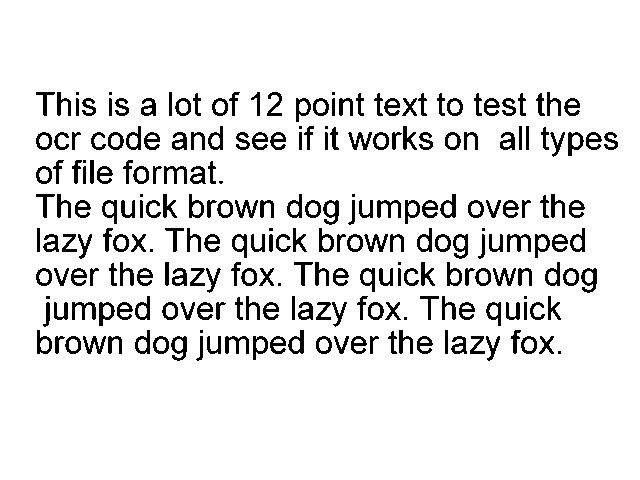# 图像文本识别：Python中的光学字符识别 (OCR)实现

2021年11月11日17:35:14 发表评论 812 次浏览

Python图像文本识别：人类只需查看图像即可轻松理解图像的文本内容。但是，对于计算机而言，情况并非如此。他们需要某种结构化的方法或算法才能理解它。这是Optical Character Recognition（OCR）的用武之地。

Python如何识别图片中的文字？光学字符识别是检测图像上的文本内容并将其转换为机器编码文本的过程，我们可以在 Python（或任何编程语言）中将其作为字符串变量访问和操作。在本教程中，我们将使用Tesseract 库来做到这一点。

Tesseract 库包含一个 OCR 引擎和一个命令行程序，所以它与 Python 无关，请按照他们的官方指南进行安装，因为它是本教程的必备工具。

tesseract 的最新稳定版本是 4，它使用新的基于循环神经网络 (LSTM)的 OCR 引擎，专注于线识别。

Python ORC识别照片中的文本示例 - 让我们开始吧，你需要安装：

• Tesseract-OCR 引擎（遵循他们的操作系统指南）。
• pytesseract 包装模块使用：`pip3 install pytesseract`复制
• 本教程的其他实用模块：`pip3 install numpy matplotlib opencv-python pillow`复制

``````import pytesseract
import cv2
import matplotlib.pyplot as plt
from PIL import Image``````我将其命名为“test.png”并将其放在当前目录中，让我们加载此图像：

``````# read the image using OpenCV
# or you can use Pillow
# image = Image.open("test.png")``````

Python如何识别图片中的文字？你可能会注意到，你可以使用OpenCV或Pillow加载图像，我更喜欢使用OpenCV，因为它使我们能够使用实时摄像头。

``````# get the string
string = pytesseract.image_to_string(image)
# print it
print(string)``````

Python图像文本识别：image_to_string()函数完全符合你的预期，它将包含的图像文本转换为字符，让我们看看结果：

``````This is a lot of 12 point text to test the
ocr code and see if it works on all types
of file format.

The quick brown dog jumped over the
lazy fox. The quick brown dog jumped
over the lazy fox. The quick brown dog
jumped over the lazy fox. The quick
brown dog jumped over the lazy fox.``````

``````# make a copy of this image to draw in
image_copy = image.copy()
# the target word to search for
target_word = "dog"
# get all data from the image
data = pytesseract.image_to_data(image, output_type=pytesseract.Output.DICT)``````

Python ORC识别照片中的文本示例：所以我们要在文本文档中搜索单词“dog”，我们希望输出数据是结构化的而不是原始字符串，这就是为什么我将output_type传递为字典的原因，这样我们就可以轻松获取每个单词的数据（你可以打印数据字典以查看输出的组织方式）。

``````# get all occurences of the that word
word_occurences = [ i for i, word in enumerate(data["text"]) if word.lower() == target_word ]``````

``````for occ in word_occurences:
# extract the width, height, top and left position for that detected word
w = data["width"][occ]
h = data["height"][occ]
l = data["left"][occ]
t = data["top"][occ]
# define all the surrounding box points
p1 = (l, t)
p2 = (l + w, t)
p3 = (l + w, t + h)
p4 = (l, t + h)
# draw the 4 lines (rectangular)
image_copy = cv2.line(image_copy, p1, p2, color=(255, 0, 0), thickness=2)
image_copy = cv2.line(image_copy, p2, p3, color=(255, 0, 0), thickness=2)
image_copy = cv2.line(image_copy, p3, p4, color=(255, 0, 0), thickness=2)
image_copy = cv2.line(image_copy, p4, p1, color=(255, 0, 0), thickness=2)``````

``````plt.imsave("all_dog_words.png", image_copy)
plt.imshow(image_copy)
plt.show()``````

Python如何识别图片中的文字？看看结果：